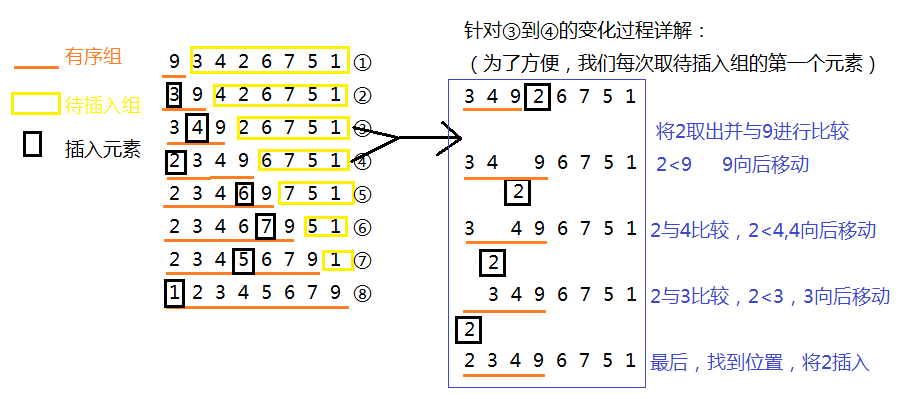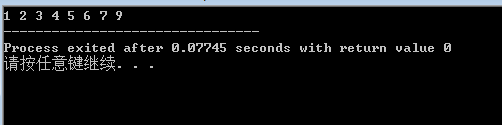插入排序算法详解及实现#include<stdio.h>
void InsertionSort(int *num,int n)
{
int i = 0;
int j = 0;
int tmp = 0;
for(i = 1;i<n;i++)
{
tmp = num[i];//从待插入组取出第一个元素。
j = i-1; //i-1即为有序组最后一个元素（与待插入元素相邻）的下标
while(j>=0&&tmp<num[j])  //注意判断条件为两个，j>=0对其进行边界限制。第二个为插入判断条件
{
num[j+1] = num[j];//若不是合适位置，有序组元素向后移动
j--;
}
num[j+1] = tmp;//找到合适位置，将元素插入。
}
}
int main()
{
int i = 0;
int num={9,3,4,2,6,7,5,1};
InsertionSort(num,8);
/*这个函数必须知道元素的个数，所以将元素个数传入。
有心者可以在函数内部用sizeof求出元素个数 */
for(i=0;i<8;i++)
{
printf("%d ",num[i]);
}
return 0;
}